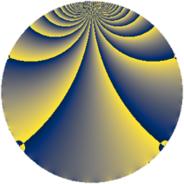# Properties

 Label 896.2.eLevel $896$ Weight $2$ Character orbit 896.e Rep. character $\chi_{896}(447,\cdot)$ Character field $\Q$ Dimension $32$ Newform subspaces $7$ Sturm bound $256$ Trace bound $7$

# Related objects

## Defining parameters

 Level: $$N$$ $$=$$ $$896 = 2^{7} \cdot 7$$ Weight: $$k$$ $$=$$ $$2$$ Character orbit: $$[\chi]$$ $$=$$ 896.e (of order $$2$$ and degree $$1$$) Character conductor: $$\operatorname{cond}(\chi)$$ $$=$$ $$56$$ Character field: $$\Q$$ Newform subspaces: $$7$$ Sturm bound: $$256$$ Trace bound: $$7$$ Distinguishing $$T_p$$: $$3$$, $$5$$, $$11$$, $$31$$

## Dimensions

The following table gives the dimensions of various subspaces of $$M_{2}(896, [\chi])$$.

Total New Old
Modular forms 144 32 112
Cusp forms 112 32 80
Eisenstein series 32 0 32

## Trace form

 $$32q - 32q^{9} + O(q^{10})$$ $$32q - 32q^{9} + 32q^{25} - 32q^{49} - 64q^{57} - 32q^{81} + O(q^{100})$$

## Decomposition of $$S_{2}^{\mathrm{new}}(896, [\chi])$$ into newform subspaces

Label Dim. $$A$$ Field CM Traces $q$-expansion
$$a_2$$ $$a_3$$ $$a_5$$ $$a_7$$
896.2.e.a $$4$$ $$7.155$$ $$\Q(\zeta_{12})$$ None $$0$$ $$0$$ $$-4$$ $$-8$$ $$q+(\zeta_{12}-\zeta_{12}^{2})q^{3}+(-1-\zeta_{12}^{3})q^{5}+\cdots$$
896.2.e.b $$4$$ $$7.155$$ $$\Q(\zeta_{12})$$ None $$0$$ $$0$$ $$-4$$ $$8$$ $$q+(\zeta_{12}-\zeta_{12}^{2})q^{3}+(-1-\zeta_{12}^{3})q^{5}+\cdots$$
896.2.e.c $$4$$ $$7.155$$ $$\Q(\sqrt{-2}, \sqrt{-3})$$ None $$0$$ $$0$$ $$0$$ $$-8$$ $$q+\beta _{1}q^{3}-\beta _{3}q^{5}+(-2-\beta _{2})q^{7}+q^{9}+\cdots$$
896.2.e.d $$4$$ $$7.155$$ $$\Q(\sqrt{-2}, \sqrt{-3})$$ None $$0$$ $$0$$ $$0$$ $$8$$ $$q+\beta _{1}q^{3}+\beta _{3}q^{5}+(2+\beta _{2})q^{7}+q^{9}+\cdots$$
896.2.e.e $$4$$ $$7.155$$ $$\Q(\zeta_{12})$$ None $$0$$ $$0$$ $$4$$ $$-8$$ $$q+(\zeta_{12}-\zeta_{12}^{2})q^{3}+(1+\zeta_{12}^{3})q^{5}+\cdots$$
896.2.e.f $$4$$ $$7.155$$ $$\Q(\zeta_{12})$$ None $$0$$ $$0$$ $$4$$ $$8$$ $$q+(\zeta_{12}-\zeta_{12}^{2})q^{3}+(1+\zeta_{12}^{3})q^{5}+\cdots$$
896.2.e.g $$8$$ $$7.155$$ 8.0.2517630976.5 $$\Q(\sqrt{-14})$$ $$0$$ $$0$$ $$0$$ $$0$$ $$q+\beta _{4}q^{3}-\beta _{2}q^{5}-\beta _{3}q^{7}+(-3+\beta _{1}+\cdots)q^{9}+\cdots$$

## Decomposition of $$S_{2}^{\mathrm{old}}(896, [\chi])$$ into lower level spaces

$$S_{2}^{\mathrm{old}}(896, [\chi]) \cong$$ $$S_{2}^{\mathrm{new}}(56, [\chi])$$$$^{\oplus 5}$$$$\oplus$$$$S_{2}^{\mathrm{new}}(224, [\chi])$$$$^{\oplus 3}$$$$\oplus$$$$S_{2}^{\mathrm{new}}(448, [\chi])$$$$^{\oplus 2}$$